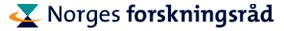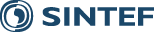Overview of Lectures

### Sunday March 7:

15:30 - 19:30, Larson: Crash course in finite elements
We give a basic introduction to finite element methods including:
• Construction of piecewise polynomial function spaces, interpolation and projection operators, and approximation properties.
• Finite element discretization of elliptic problems and derivation of linear system of equations, applications to heat conduction and elasticity.
• Basic a priori error estimates.
• Stabilized finite elements with applications to flow.
• Other finite element methods, for instance mixed and discontinuous Galerkin methods.

### Monday March 8:

09:05 - 10:45, Larson: Basic techniques of a posteriori error estimation.
We present a posteriori error estimates in the energy norm based on residuals, recovery, and different local problems; lower bounds and adaptive strategies; mesh refinement techniques; and examples including elliptic problems and elasticity.

15:00 - 16:30, Larson: Convergence of adaptive algorithms
We present recent theoretical results on the convergence of adaptive methods.

17:00 - 18:30, Larson: Goal oriented a posteriori error estimates based on duality techniques.
We introduce the basic idea of using the solution of a dual problem to express the error in a goal quantity in terms of the residual and present a few different techniques deriving a posteriori error estimates. Next we discuss various ways to implement the estimates. Several examples of applications are presented. Finally, we discuss post processing procedures based on duality techniques.

### Tuesday March 9:

09:00 - 10:30, Larson: Time dependent problems
A posteriori error estimation for time-dependent problems including applications to heat conduction, flow, and finance.

15:30 - 17:00, Rannacher: Practical aspects of the Dual Weighted Residual (DWR) method.
We discuss some practical aspects of the approximate evaluation of weighted a posteriori error estimators and the resulting mesh adaptation strategies. This includes mesh optimization, anisotropic mesh adaptation and h/p adaptation.

### Wednesday March 10:

09:00 - 10:30, Larson: Submodeling, domain decomposition, and composite grids.
A posteriori error estimation for submodeling or local-global methods and domain decomposition algorithms. We also present estimates for discontinuous Galerkin methods and their application to composite (nonmatching) grids.

15:00 - 16:30, Rannacher: Nonstandard linear problems.
The particular feature of the DWR method is its applicability to situations in which the underlying mathematical model lacks quantitative coercivity properties. As illustrative examples, we discuss the adaptive solution of the radiative transfer equation and the acoustic wave equation.

17:00 - 18:30, Rannacher: Nonlinear problems
We introduce a general paradigm for residual-based adaptivity in the Galerkin approximation of nonlinear variational problems. This provides the abstract framework for the later application to various types of nonlinear problems in solid and fluid mechanics including also eigenvalue and optimal control problems.

### Thursday March 11:

09:00 - 10:30, Rannacher: Application in solid mechanics.
We discuss the use of the DWR method for the finite element solution of problems in linear elasticity and in elasto-plasticity. This includes the treatment of incompressible material which prepares for fluid mechanical applications.

15:00 - 16:30, Rannacher: Application in fluid mechanics.
We discuss the use of the DWR method for the finite element approximation of models for viscous incompressible and compressible flow including also chemical reactions. The examples presented include the computation of drag and lift coefficients in two- and three-dimensional pipe flows, the mean Nusselt number in a heat exchanger, the line-averages of species concentration in a flow reactor, and the mean temperature in a methane flame.

17:00 - 18:30, Rannacher: Model adaptivity.
The concept of residual-based adaptivity used in the DWR method can be extended to the hierarchical approximation or enrichment of mathematical models. At first, the underlying idea will be illustrated for simple model cases, and then a more advanced application to chemically reactive flow will be presented. This is closely related to parameter estimation which will be treated in the last lecture.

### Friday March 12:

09:00 - 10:30, Rannacher: Application in optimal control.
We discuss the use of the DWR method in the finite element discretization of optimal control problems. As examples, we consider boundary control in heat transfer and drag minimization in viscous flow.

11:00 - 12:30, Rannacher: Application in parameter estimation.
We discuss the use of the DWR method in the finite element discretization of parameter estimation and eigenvalue problems. This will be illustrated by several examples ranging from the determination of diffusion coefficients to problems in hydrodynamic stability. The lecture will close with an outlook to further developments and open problems.

Published October 20, 2010